CBSE Class 12 Sample Paper for 2023 Boards

Class 12
Solutions of Sample Papers and Past Year Papers - for Class 12 Boards

## Find the points of local maximum/local minimum, if any, in the interval (0, 12) as well as the points of absolute maximum/absolute minimum in the interval [0, 12]. Also, find the corresponding local maximum/local minimum and the absolute maximum/absolute minimum values of the function.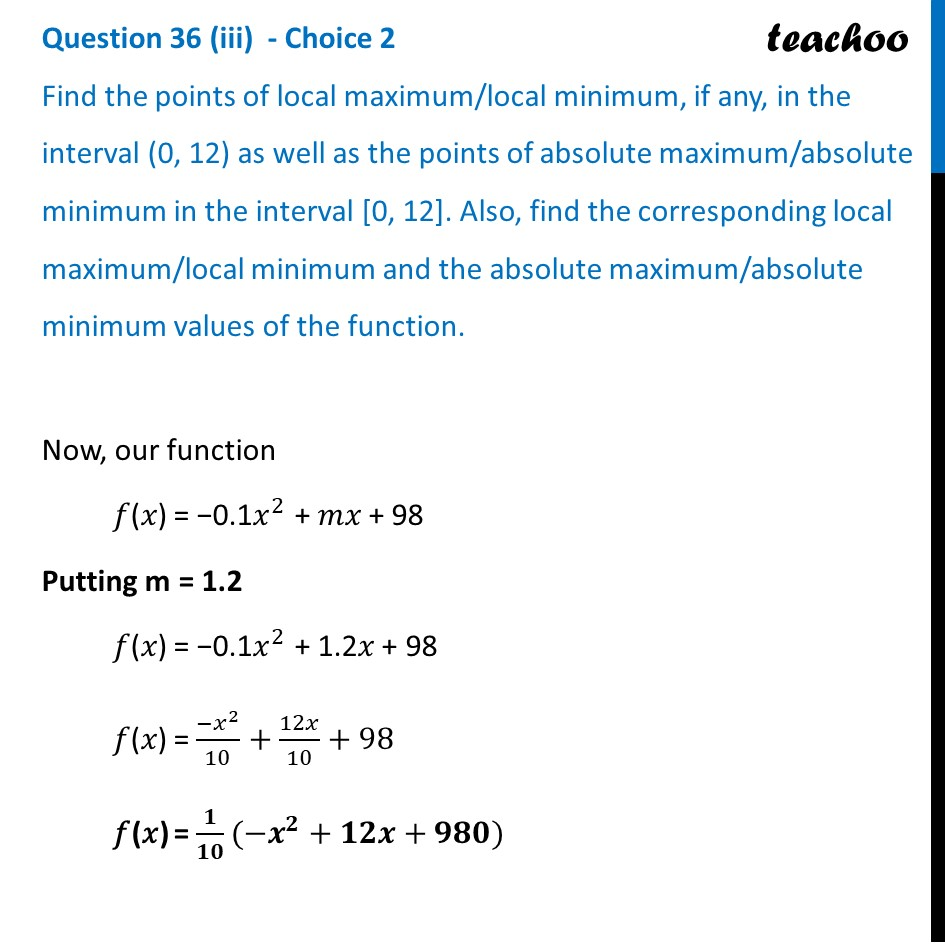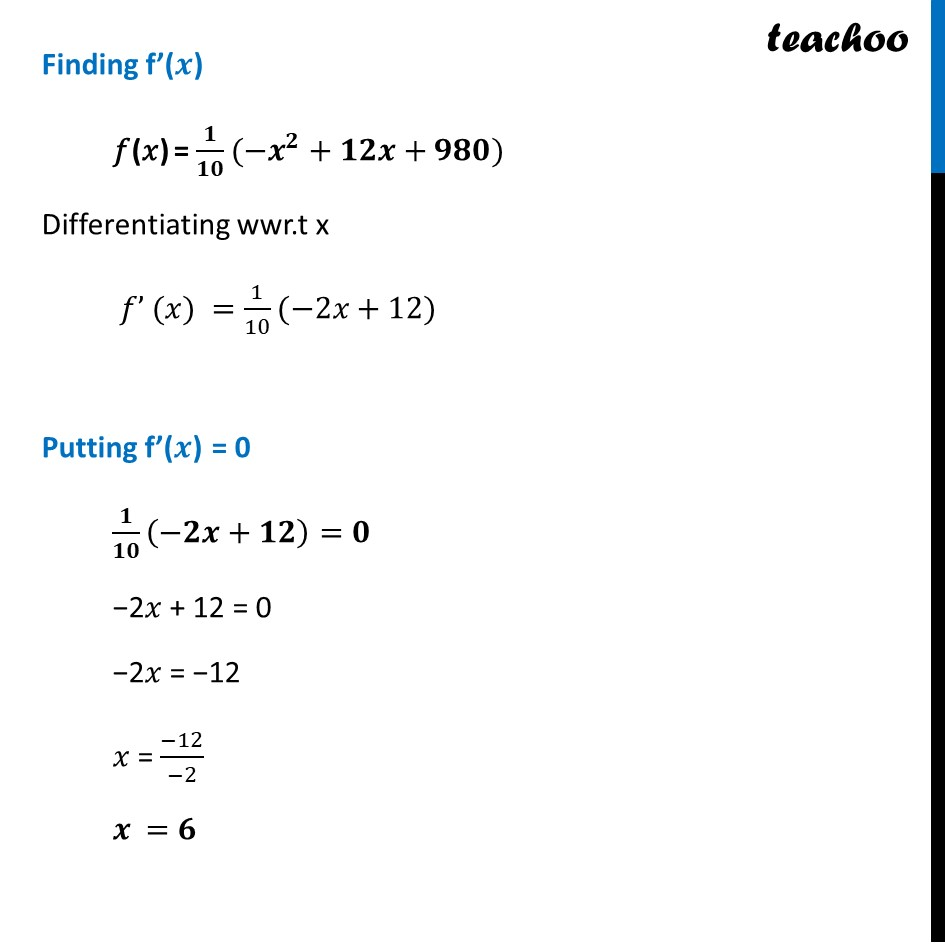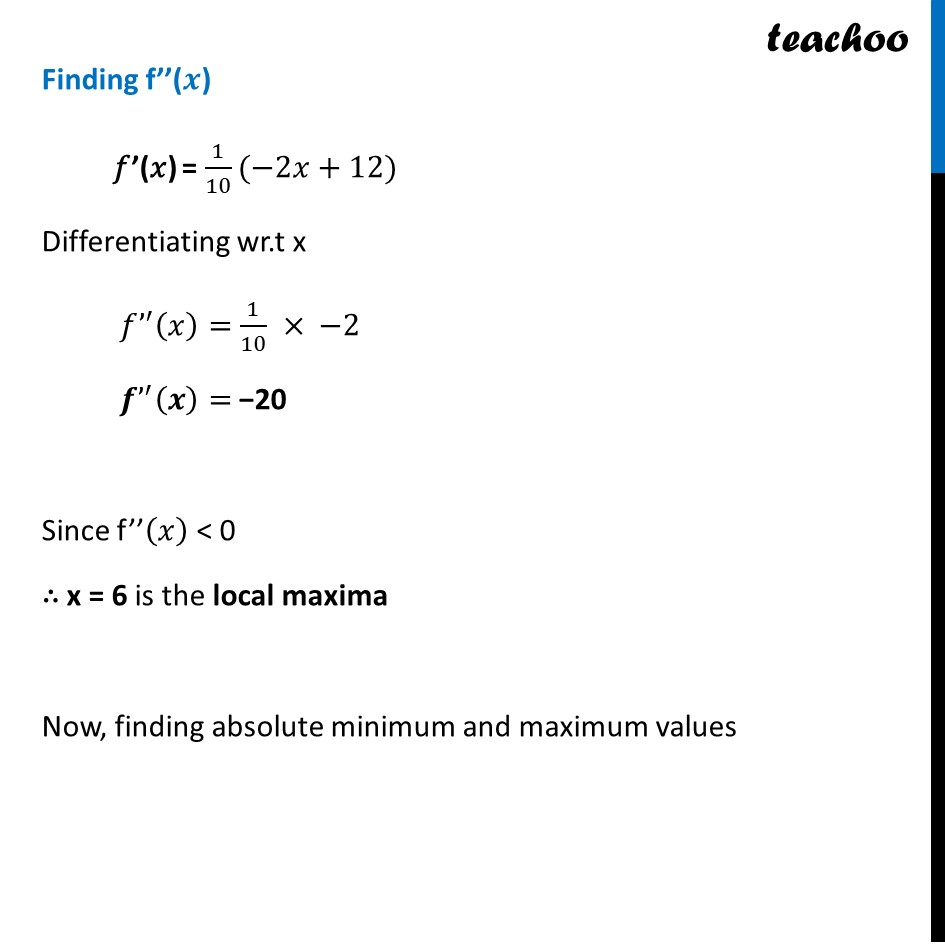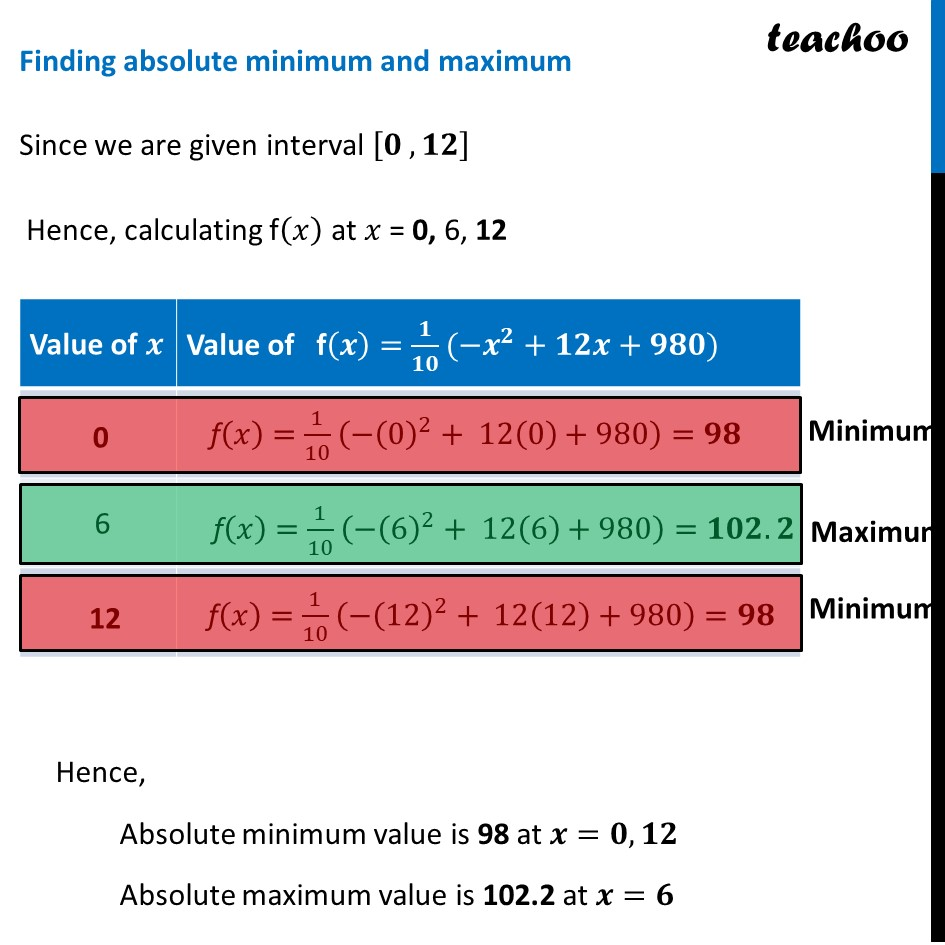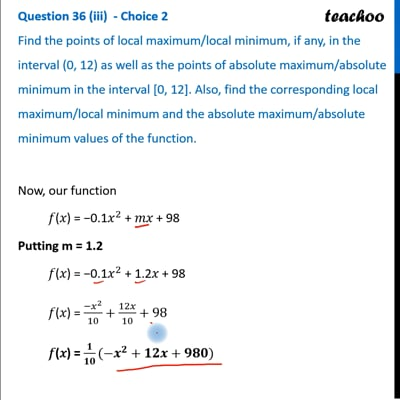This video is only available for Teachoo black users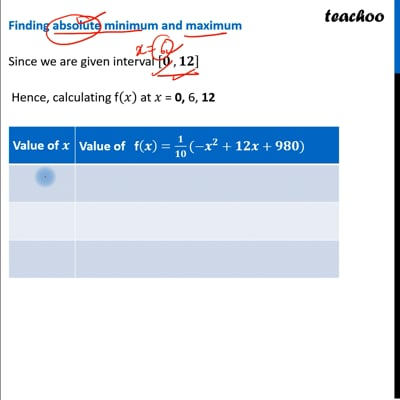This video is only available for Teachoo black users

Learn in your speed, with individual attention - Teachoo Maths 1-on-1 Class

### Transcript

Question 36 (iii) - Choice 2 Find the points of local maximum/local minimum, if any, in the interval (0, 12) as well as the points of absolute maximum/absolute minimum in the interval [0, 12]. Also, find the corresponding local maximum/local minimum and the absolute maximum/absolute minimum values of the function.Now, our function 𝑓(𝑥) = −0.1𝑥^2 + 𝑚𝑥 + 98 Putting m = 1.2 𝑓(𝑥) = −0.1𝑥^2 + 1.2𝑥 + 98 𝑓(𝑥) = 〖−𝑥〗^2/10+12𝑥/10+98 𝑓(𝑥) = 𝟏/𝟏𝟎(−𝒙^𝟐+𝟏𝟐𝒙+𝟗𝟖𝟎) Finding f’(𝒙) 𝑓(𝑥) = 𝟏/𝟏𝟎(−𝒙^𝟐+𝟏𝟐𝒙+𝟗𝟖𝟎) Differentiating wwr.t x 𝑓’ (𝑥) =1/10(−2𝑥+12) Putting f’(𝒙) = 0 𝟏/𝟏𝟎 (−𝟐𝒙+𝟏𝟐)=𝟎 −2𝑥 + 12 = 0 −2𝑥 = −12 𝑥 = (−12)/(−2) 𝒙 =𝟔 Finding f’(𝒙) 𝑓(𝑥) = 𝟏/𝟏𝟎(−𝒙^𝟐+𝟏𝟐𝒙+𝟗𝟖𝟎) Differentiating wwr.t x 𝑓’ (𝑥) =1/10(−2𝑥+12) Putting f’(𝒙) = 0 𝟏/𝟏𝟎 (−𝟐𝒙+𝟏𝟐)=𝟎 −2𝑥 + 12 = 0 −2𝑥 = −12 𝑥 = (−12)/(−2) 𝒙 =𝟔 Finding f’’(𝒙) 𝑓’(𝑥) = 1/10(−2𝑥+12) Differentiating wr.t x 𝑓’^′ (𝑥)=1/10 × −2 𝒇’^′ (𝒙)= −20 Since f’’(𝑥) < 0 ∴ x = 6 is the local maxima Now, finding absolute minimum and maximum values Finding absolute minimum and maximum Since we are given interval [𝟎 , 𝟏𝟐] Hence, calculating f(𝑥) at 𝑥 = 0, 6, 12Hence, Absolute minimum value is 98 at 𝒙=𝟎, 𝟏𝟐 Absolute maximum value is 102.2 at 𝒙=𝟔